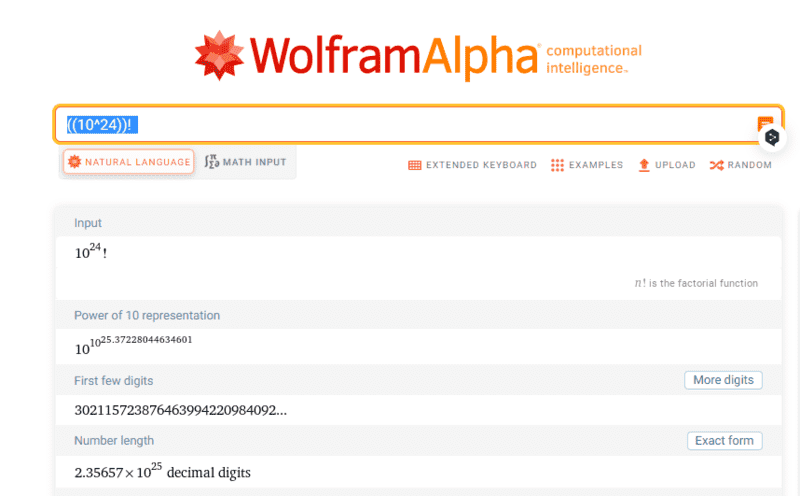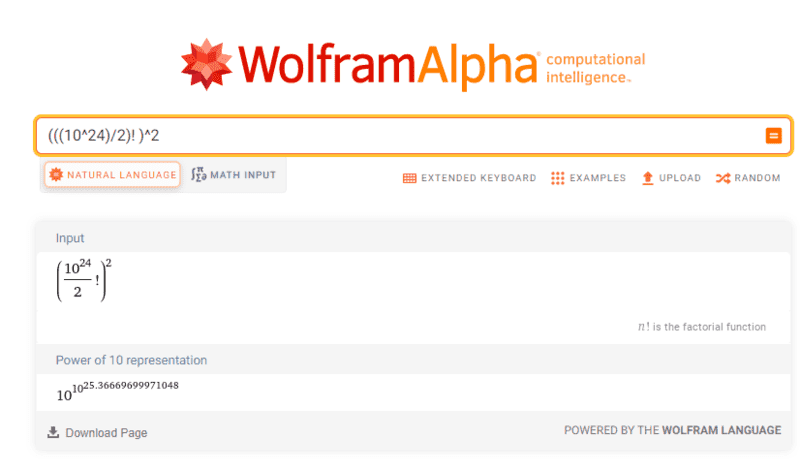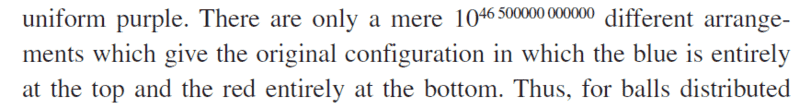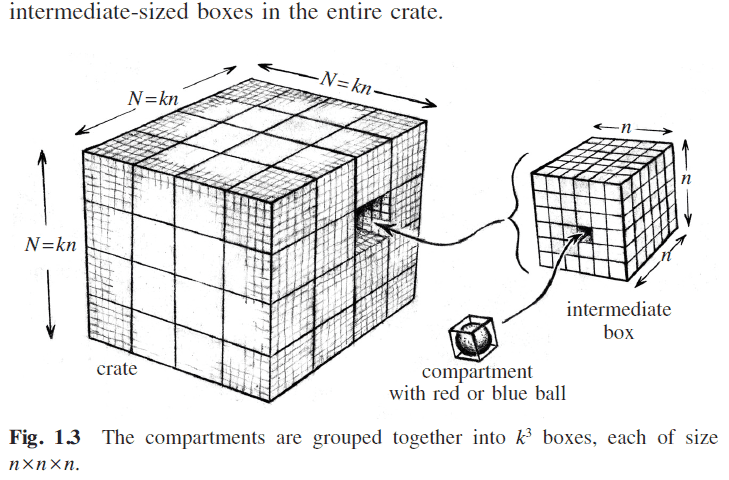# State counting (Entropy)

• I
matsu
In the book "Cycles of Time" by Roger Penrose, there is a part of the explanation of entropy that I don't understand.
There are 10^24 balls, half of which are red and the other half blue.
The model is to arrange the balls in a cube with 10^8 balls on each edge.
It also divides the cube into smaller cubes of 10^5 on a edge, and defines a cube as uniformly purple
if the blue/red ratio in the cube is between 0.999 and 1.001.
Then comes the description of the values A and B below, which are approximate values that
are unclear how they were calculated.

(Value A)Number of states in which the entire 10^24 cube is uniformly purple: 10^23,570,00
0,000,000,000,000,000,000,000
(Value B)Number of states where the top half of the 10^24 cube is blue and the bottom half
is red: 10^46,500,000,000,000,000,000

I guess that the value A would be the value of (10^24)! but I have no idea how value B is calculated.
Can anyone give me a hint or help?

Note: The same question was asked in a previous thread, but the value B was not resolved.

Homework Helper
Can you write out the Stirling expression evaluation for the A number?

Should be something with log( 1024 ! )

Then do the same for half that number and take the square....

 two things:
1. You have three zeroes too many. In the book he has
23 570000 000000 000000 000000, not
23,570,000,000,000,000,000,000,000,000

2. My recipe doesn't seem to work-- have to dig a little deeper

## \ ##

Last edited:
•matsu
matsu

1. You have three zeroes too many. In the book he has
23 570000 000000 000000 000000, not
23,570,000,000,000,000,000,000,000,000
Sorry, I wrote it wrong.
2.357 * 10^25 is the correct value A.

Can you write out the Stirling expression evaluation for the A number?

I did not use Stirling's approximation, but used WolframAlpha to get the approximation below.2. My recipe doesn't seem to work-- have to dig a little deeper
I did the same calculation but it did not work.
The number is too large for the value B.Could you please let me know if there is any progress?

Homework Helper
I did not use Stirling's approximation
Why not ? Missed a nice opportunity ...
##N\log N - N = 10^{ 2.2357e25}\ ## for ##N=1e24## (number A)

With ##N=5e23## I get ##N\log N - N = 10^{1.163e25}\ ## and the square of that is ##10^{2.326e25}## which is a factor ##3.01e23## lower. Enormously lower, but it gets us nowhere near the ##10^{4.65e19}##

I did the same calculation but it did not work.
So now we're both stuckand we need some help. I'll ask around.

##\ ##

•matsu
matsu
Why not ? Missed a nice opportunity ...
##N\log N - N = 10^{ 2.2357e25}\ ## for ##N=1e24## (number A)

With ##N=5e23## I get ##N\log N - N = 10^{1.163e25}\ ## and the square of that is ##10^{2.326e25}## which is a factor ##3.01e23## lower. Enormously lower, but it gets us nowhere near the ##10^{4.65e19}##
Thanks for the clear expression about the Stirling approximation.
I can now do the calculations myself.

So now we're both stuckand we need some help. I'll ask around.
I appreciate your help so much!

Homework Helper
...
and the square of that is ##10^{2.326e25}## which is a factor ##3.01e23## lower. Enormously lower, but it gets us nowhere near the ##10^{4.65e19}##
It's even lower: I posted the base 10 logarithm. The factor itself is ##10^{\,10^{\,3.01\times 10^{23}}}\ ## as @mfb pointed out.

So now we're both stuckand we need some help. I'll ask around.
No-progress report: so far I have not found a way to come closer to the
##10^{\,4.65\times 10^{19}}\ ## Penrose stateswhich is as good as zero.

##\ ##

Philip Koeck
(Value A)Number of states in which the entire 10^24 cube is uniformly purple: 10^23,570,00
0,000,000,000,000,000,000,000

I guess that the value A would be the value of (10^24)!
(10^24)! would be all the ways of placing 10^24 balls in the cube, wouldn't it?
But that would include configurations where a large part of the cube is just blue or just red, not only uniformly purple configurations.

Philip Koeck
(Value B)Number of states where the top half of the 10^24 cube is blue and the bottom half
is red: 10^46,500,000,000,000,000,000
For B I suggest ((0.5 x 10^24)!)^2

Homework Helper
For B I suggest ((0.5 x 10^24)!)^2
As worked out in #4.
(with a slight correction in #6)

But Penrose B is hideously smaller....

## \ ##

•Philip Koeck
Philip Koeck
As worked out in #4.
(with a slight correction in #6)

But Penrose B is hideously smaller....

## \ ##
Ah, I missed that you'd already worked out B.

I can't see any other way to calculate B. Maybe Penrose just got it wrong?

It's also obvious that (1024)! can't be right for A, isn't it?

Homework Helper
It's also obvious that (1024)! can't be right for A, isn't it?
True, but the point is to show that subtracting not-purple leaves us with the same number -- at least down to the first 23 or 24 decimals.

##\ ##

•Philip Koeck
Philip Koeck
True, but the point is to show that subtracting not-purple leaves us with the same number -- at least down to the first 23 or 24 decimals.

##\ ##
What about looking at something simpler: If we require the smaller cubes to have exactly the ratio 1 between blue and red balls we should get a value A' that's smaller than Penrose's A:

A' = ((0.5 x 1024)!)2 x ((1015)! / ((0.5 x 1015)! (0.5 x 1015)!))1 000 000 000

Last edited:
Homework Helper
Don't understand how this A' comes about.

Penrose introduces intermediate-sized boxes (your 'smaller cubes', I assume)and has ##N = 10^8,\ k = 10^3,\ n = 10^5##. 'purple' means red/blue in ALL intermediate boxes is between 0.999 and 1.001. He finds (without explaining) there are ##10^{10^{ 2.357\times 10^{25}}} \ ## different arrangements are 'purple' . Our number A .

As it happens, ##\left ( 10^{24} \right)\,! ## -- the way to arrange 1024 balls on 1024 positions -- is also this number. Well... the whole treatment is supposed to explain (make plausible) that A is extremely close to ##\left ( 10^{24} \right)\,! ## . We all know A ##<< \left ( 10^{24} \right)\,! ## of course, but the whole story is aimed at how much smaller.

Penrose then does NOT work out a number for not-uniformly-all-'purple' configurations, but instead states (see #6) a number for 'all blue at the top' that is much too small (we found ##10^{10^{2.326\times 10^{25}}}\ ## in #6 -- meaning the number for not-uniformly-all-'purple' configurations is frighteningly close to ##\left ( 10^{24} \right)\,! ## as well)

(and once more: sorry for the many mishaps due to boggling mind)​

So, for me the inevitable conclusion is that Penrose does not do a good job here.

a while ago I proposed
Start with 32 white balls and 32 black balls in a 4x4x4 box ...
but this too doesn't help us to end up at the ##10^{4.65\times 10^{19}}## in the book -- sorry @matsu !

##\ ##

votingmachine
(Value A)Number of states in which the entire 10^24 cube is uniformly purple: 10^23,570,00
0,000,000,000,000,000,000,000
(Value B)Number of states where the top half of the 10^24 cube is blue and the bottom half
is red: 10^46,500,000,000,000,000,000

I guess that the value A would be the value of (10^24)! but I have no idea how value B is calculated.
Can anyone give me a hint or help?
It would not be 10^24)! Because they are not 10^24 different balls, but are two groups of 0.5x10^24 equivalent balls.

I'm having trouble working thru it, but consider the simplified 2x2 matrix. There are 6 possible states:
r|r r|b r|b b|r b|r b|b
b|b b|r r|b b|r r|b r|r

4! would be 24 possible states.

I was pondering a 2x2x2 cube with 8 positions. and the number of states is quite large. But again, smaller than the factoral.

It was not clear to me whether the blue-red split between top and bottom was based on the 0.999 to 1.001 is purple, else blue or red.

Philip Koeck
It would not be 10^24)! Because they are not 10^24 different balls, but are two groups of 0.5x10^24 equivalent balls.

I'm having trouble working thru it, but consider the simplified 2x2 matrix. There are 6 possible states:
r|r r|b r|b b|r b|r b|b
b|b b|r r|b b|r r|b r|r

4! would be 24 possible states.

I was pondering a 2x2x2 cube with 8 positions. and the number of states is quite large. But again, smaller than the factoral.

It was not clear to me whether the blue-red split between top and bottom was based on the 0.999 to 1.001 is purple, else blue or red.
The way I read it Penrose is considering distinguishable balls. So you get a new state by swapping two balls of the same color.
Otherwise B would be 1.

•BvU
Philip Koeck
Don't understand how this A' comes about.

Penrose introduces intermediate-sized boxes (your 'smaller cubes', I assume)
Not sure it's right, but here's my thinking:

There are 1 000 000 000 smaller cubes (intermediate boxes) in the big box.

First I fill the lower halves (exactly) of all the smaller cubes with red and the upper halves with blue balls.
For this there are ((0.5 x 1024)!)2 possibilities, the same as our value for B.

Now I have to take into account that it doesn't matter how the red and blue balls are placed in the smaller cubes as long as they are equal in number in each box.
I have to multiply with the number of permutations within each smaller box, but avoid re-counting the permutations I've already considered (the ones only involving balls of the same color).
For each smaller box that gives a factor (1015)!/((0.5 x 1015)!)2 and there are 1 000 000 000 smaller boxes.

Last edited:
votingmachine
The way I read it Penrose is considering distinguishable balls. So you get a new state by swapping two balls of the same color.
Otherwise B would be 1.
Hmm. I was reading it as a model of say air, where there are indistinguishable nitrogen molecules and indistinguishable oxygen molecules, and then what is the probability of even a slight deviation from a perfect mix.

And the easiest approximation I thought of was using the idea of replacing each ball selection with a coin flip, and then using the binomial distribution for determining the states outside the very nearly 1:1 ratio. But that is a different entropy problem.

•Philip Koeck
Philip Koeck
Hmm. I was reading it as a model of say air, where there are indistinguishable nitrogen molecules and indistinguishable oxygen molecules, and then what is the probability of even a slight deviation from a perfect mix.
I don't really know what Penrose is modelling, but I don't think it's a good model of a gas mixture.
In the model there is only one available state per particle and each state can only take one particle.
That's why there is only one way of putting all the red balls in the lower half and all the blue in the upper half if balls of the same color are indistinguishable from each other. B = 1 for indistinguishable balls.

In a gas there are always many more states than atoms (or molecules).
That means that, for a mixture of two gases, there are very many ways of putting all atoms of one kind in one half of a container even if atoms of the same kind are indistinguishable from each other.

Homework Helper
Hmm. I was reading it as a model of say air, where there are indistinguishable nitrogen molecules and indistinguishable oxygen molecules, and then what is the probability of even a slight deviation from a perfect mix.

And the easiest approximation I thought of was using the idea of replacing each ball selection with a coin flip, and then using the binomial distribution for determining the states outside the very nearly 1:1 ratio. But that is a different entropy problem.
The story is about paint, the treatment is as if it's about a solid. Distinguishable balls, half of them blue, the other half red.
A coin flip is supposed to have the same probability every time -- you could end up with all blue.

So ##M## coin flips yield ##P = \displaystyle \left (1\over 2\right )^M## probability of all heads. If ##M = {1\over 2}N\ ## then ##\ \log P = -{1\over 2}N\log 2## .

Placing ##N## balls in ##N## locations can be done in ##A' = N\,!## ways.

Placing ##{1\over 2}N## blue balls ##{1\over 2}N## locations (say the top half of the cube) can be done in ##C = \left ( {1\over 2}N\right ) \,!## ways. All the red ones in the other half of the cube idem.

So ratio ##Q## (top half blue and bottom half red)/(totally random) is ##C^2/A'##.

Stirling: ##\ \log A'= N\log N - N \ ## and ##\ \log C= {1\over 2}N\log {1\over 2}N - {1\over 2}N \ ## so that
##\log Q = N\left(\log {1\over 2}N - \log N\right )= -N\log 2 \quad ##( with thanks to @mfb )

Meaning ##Q=P^2\ ##: with coin flips it's a lot easier than with ballsAll this is slightly (well...) repetitive and doesn't get us anywhere in understanding how Penrose messes up, I'm afraid##\ ##

•Philip Koeck
votingmachine
N! makes sense to me now. To calculate the number of states with the bottom uniformly red, you could calculate the probability and multiply by the number of states. I can't see how the calculation would go but the sequence is:
1st ball probability: 0.5N / N
2nd ball probability: (0.5N-1) / N-1
3rd ball probability: (0.5N-2) / N-2

The probabilities would be multiplied for the total

Someone proposed a simplification with a 4x4x4 cube and 32 balls.

The probability would then be:
Product (i from 0 to 15) of (0.5N-i) / N
Product (i from 0 to 15) of (16-i) / (32-i)
16/32 x 15/31 x ... x 1/17 = 16! / (32! / 16!) = (16!^2) / 32!

Mutiply that times 32!
[(16!^2) / 32!] x 32! = (16!^2)

I think it would generalize to:

(0.5N! ^2) = number of states with half one color

I caught one math error as I re-read this ... it is entirely possible there is another in plane sight. I think the principle of calculating the number of states from the probability times the total number of states would work though.

EDIT: a 4x4x4 cube is 64 balls. I missed that math error.
EDIT-EDIT: I see now that this was worked out in post #7.

Last edited:
Philip Koeck
What about looking at something simpler: If we require the smaller cubes to have exactly the ratio 1 between blue and red balls we should get a value A' that's smaller than Penrose's A:

A' = ((0.5 x 1024)!)2 x ((1015)! / ((0.5 x 1015)! (0.5 x 1015)!))1 000 000 000
An idea:

Assuming my value A' for exactly purple is correct we can allow for deviations from exactly purple like this.
There are S different shades of purple that fit the definition of "uniformly purple".
S should be 0.5 x 1012 in the example, right?
If each of them approximately has the same statistical weight (A') then we can get A from

A ≈ A' S1 000 000 000

Not sure, is that right?

Last edited: